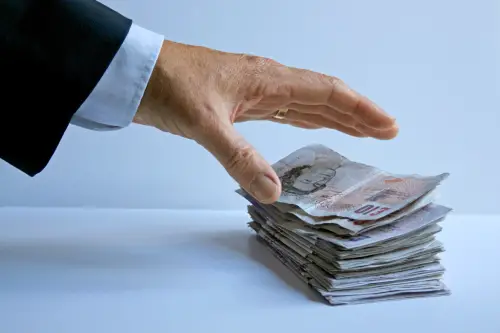Income, Expenses and Retained Earnings Question

by Roderick Merritt
(Goldsboro, NC, USA)

Q: Andersen's Nursery has sales of \$318,400, cost of \$199,400, depreciation expense of \$28,600, interest expense of \$1,000, and a tax rate of 34%. The firm paid out \$16,500 in dividends.

What is the addition to retained earnings?A:
Great question Roderick and a good example of income and profits and their distribution for a company.

Here's the solution (income statement):

Sales \$318,400
Less: Cost of sales \$199,400
Gross Profit \$119,000 (\$318,400-\$199,400)
Less: Depreciation \$28,600
Less: Interest expense \$1,000
Net profit before tax \$89,400 (\$119,400 - \$28,600 - \$1,000)
Less: Tax \$30,396 (\$89,400 X 34/100)
Net profit after tax \$59,004 (\$89,400 - \$30,396)

If \$16,500 of the net profit after tax was paid out in dividends then
\$42,504 (\$59,004 - \$16,500) was left over for the retained earnings account.

Notes:
• Retained earnings is an account where a company accumulates profits (earnings) and from which dividends are paid to the owners. It is a type of reserve. Reserves are the 2nd main type of equity besides capital. Both capital (invested assets, usually cash) and reserves (accumulated profits) belong to the owner.

• Depreciation is an expense recording the gradual decrease of an asset's value over time. You can get a bunch of in-depth lessons, examples and exercises on depreciation in my basic accounting books.

• Dividends are a payout of profits (usually in the form of cash) to the owners of a company. They are like drawings but specifically for a company. Owners in a company are called shareholders.